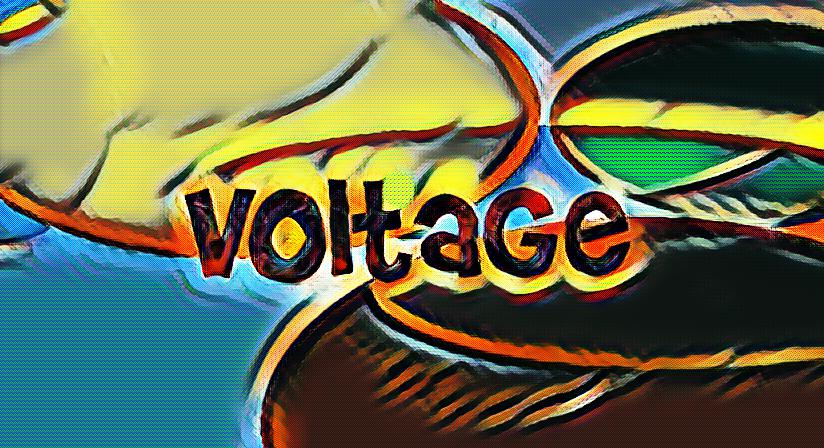# What is voltage?

Voltage is technically called the electric potential difference, and this synonym really gets at the way voltage works. Voltage is technically the difference in the electrical potential between two points. Getting even more technical, voltage is the difference between two points in a static electric field.

## Voltage, Work and Charge

Voltage is the difference between two points in a field whereby voltage is defined as the amount of work needed per unit of charge to transition a test charge between two points. A test charge can be thought of as an idealized model in which there is negligible hindrance between the work needed per unit of charge and the difference in electrical potential between two points.

Voltage is sometimes referred to by physicists as electromotive force because voltage is technically a quantifiable expression of the difference in charge between two points lying in the same electrical field. The greater the voltage in a given medium, the higher the flow of electric current there should be between a conducting medium given a certain resistance to the flow of that electrical current.

## Direct and Alternating Voltage

Direct and alternating voltage are distinguished from each other by polarity and the potential change in a polarity’s type and direction over time. Direct voltage is relatively straightforward since it maintains the same polarity over time. Alternating voltage, by contrast, reverses the polarity’s direction at certain points in time. The number of cycles per second that this polarity alters is known as the frequency.

This frequency change is measured in hertz. Terms like kilohertz and gigahertz denote higher cycles per second. An electrochemical cell deals with direct voltage whereas the aforementioned frequency changes in hertz are more related to the alternating voltage of common utility outlets. A voltmeter measures these changes.

Voltage is a key parameter of all electrical circuits. Voltage is fundamentally the pressure that forces the charged electrons to flow in an electrical circuit.